GeeksforGeeks App
Open AppBrowser
Continue

## Related Articles

• NCERT Solutions for Class 9 Maths

# Class 9 NCERT Solutions- Chapter 13 Surface Areas And Volumes – Exercise 13.8

### Question 1: Find the volume of a sphere whose radius is

(i) 7 cm (ii) 0.63 m    (Assume π =22/7)

Solution:

(i) Given: Radius of sphere, r = 7 cm

By using the formula, volume of sphere = (4/3) πr3

= (4/3)×(22/7)×(7)3

= 4312/3 cm3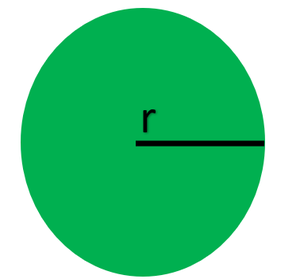Therefore, the volume of the sphere is 4312/3 cm3

(ii) Given: Radius of sphere, r = 0.63 m

By using the formula, volume of sphere = (4/3) πr3

= (4/3)×(22/7)×(0.63)3

= 1.0478 m3Therefore, the volume of the sphere is 1.05 m3 (approx).

### Question 2: Find the amount of water displaced by a solid spherical ball of diameter

(i) 28 cm (ii) 0.21 m  (Assume π =22/7)

Solution:

(i) Given: Diameter = 28 cm

Therefore, the radius, r = 28/2 cm = 14cm

By using the formula, volume of the solid spherical ball = (4/3) πr3

= (4/3)×(22/7)×(14)3

= 34496/3 cm3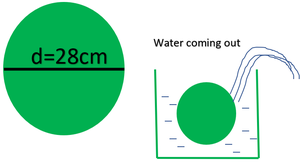Therefore, the volume of the ball is 34496/3 cm3

(ii) Given: Diameter = 0.21 m

Therefore, the radius of the ball =0.21/2 m= 0.105 m

By using the formula, volume of the ball = (4/3 )πr3

= (4/3)× (22/7)×(0.105)3

= 0.004851 m3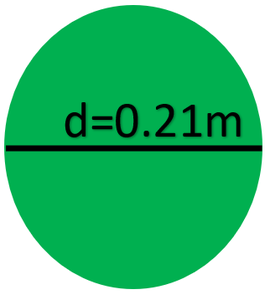Therefore, the volume of the ball = 0.004851 m3

### Question 3: The diameter of a metallic ball is 4.2cm. What is the mass of the ball, if the density of the metal is 8.9 g per cm3? (Assume π=22/7)

Solution:

Given: Diameter of a metallic ball = 4.2 cm

Therefore, the radius of the metallic ball, r = 4.2/2 cm = 2.1 cm

By using the formula, volume of the metallic ball = 4/3 πr3

= (4/3)×(22/7)×(2.1)3

= 38.808 cm3

Now by using the relationship between, density, mass, and volume,

Density = Mass/Volume

Mass = Density × volume

= (8.9×38.808) g

= 345.3912 g

Therefore, the mass of the ball is 345.39 g (approx.)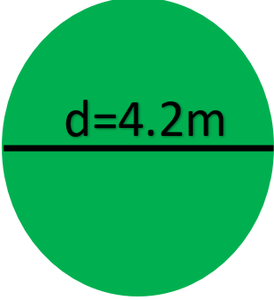### Question 4: The diameter of the moon is approximately one-fourth of the diameter of the earth. What fraction of the volume of the earth is the volume of the moon?

Solution:

Let us assume the diameter of the earth as “d”.

Therefore, the radius of the earth will be d/2

The diameter of the moon will be d/4

The radius of the moon will be d/8

Finding the volume of the moon.

By using the formula, volume of the moon = (4/3) πr

= (4/3) π (d/8)

= 4/3π(d3/512)

Finding the volume of the earth :

By using the formula, volume of the earth = (4/3) πr3

= (4/3) π (d/2)3

= 4/3π(d3/8)

The fraction of the volume of the earth to the volume of the moon

Volume of moon/volume of earth = 4/3π(d3/512)/4/3π(d3/8)

= 1/64

Therefore, the volume of moon is 1/64 th of the volume of earth.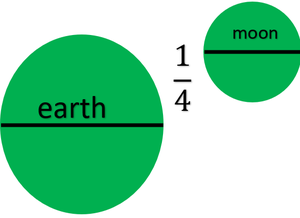### Question 5: How many liters of milk can a hemispherical bowl of diameter 10.5cm hold? (Assume π = 22/7)

Solution:

Given: Diameter of hemispherical bowl = 10.5 cm

Therefore, the radius of hemispherical bowl, r = 10.5/2 cm = 5.25 cm

By using the formula, volume of the hemispherical bowl = (2/3) πr3

= (2/3)×(22/7)×(5.25)3

= 303.1875 cm3

Therefore, the volume of the hemispherical bowl is 303.1875 cm3

Capacity of the bowl = (303.1875)/1000 L

= 0.303 liters(approx.)

Therefore, the hemispherical bowl can hold 0.303 liters of milk.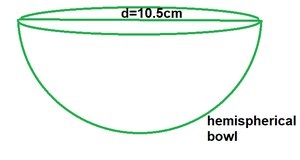### Question 6: A hemispherical tank is made up of an iron sheet 1cm thick. If the inner radius is 1 m, then find the volume of the iron used to make the tank. (Assume π = 22/7)

Solution:

Given: Inner Radius of the tank, (r) = 1m

Also, the thickness of hemispherical tank = 0.01m

Therefore, the outer Radius (R) = 1 + 0.01 = 1.01m

By using the formula, volume of the iron used in the tank = (2/3) π(R3– r3)

= (2/3)×(22/7) × (1.013– 13

= 0.06348 m3

Therefore, the volume of the iron used in the hemispherical tank is 0.06348 m3.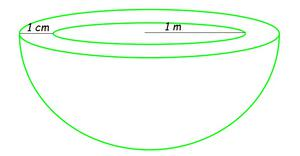### Question 7: Find the volume of a sphere whose surface area is 154 cm2. (Assume π = 22/7)

Solution:

Given: Surface area of sphere =154 cm2

Let us assume r to be the radius of a sphere.

By using the formula, Surface area of sphere = 4πr2

4πr2 = 154 cm2

r2 = (154×7)/(4 ×22)

r = 7/2

Now,

By using the formula, volume of the sphere = (4/3) πr3

= (4/3)×(22/7)×(7/2)3

= 539/3 cm3

Therefore, the volume of a sphere is 539/3 cm3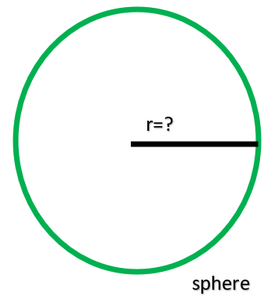### Question 8: A dome of a building is in the form of a hemisphere. From inside, it was white-washed at the cost of Rs. 4989.60. If the cost of white-washing is Rs20 per square meter, find the

(i) inside surface area of the dome

(ii) volume of the air inside the dome

(Assume π = 22/7)

Solution:

(i) Given: Cost of white-washing the dome from inside = Rs 4989.60

Also, Cost of white-washing 1m2 area = Rs 20

Therefore, CSA of the inner side of dome = 498.96/2 m2

= 249.48 m2

(ii) Let us assume the inner radius of the hemispherical dome be r.

CSA of the inner side of dome = 249.48 m2

By using the formula, CSA of a hemisphere = 2πr2

2πr2 = 249.48

2×(22/7)×r2 = 249.4

r2 = (249.48×7)/(2×22)

r2 = 39.6

r = 6.3

Hence, the radius r = 6.3 m

Volume of air inside the dome = Volume of hemispherical dome

By using the formula, volume of the hemisphere = 2/3 πr3

= (2/3)×(22/7)×(6.3)3

= 523.908 m3

= 523.9(approx.)

Therefore, the volume of air inside the dome is 523.9 m3.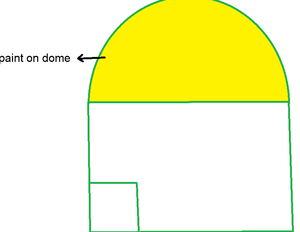### Question 9: Twenty-seven solid iron spheres, each of radius r and surface area S are melted to form a sphere with surface area S’. Find the

(i) radius r’ of the new sphere,

(ii) ratio of S and S’.

Solution:

By using the formula, volume of the solid sphere = (4/3)πr3

Therefore, the volume of twenty-seven solid sphere = 27×(4/3)πr3 = 36 πr3

(i) Given: new solid iron sphere radius = r’

By using the formula, volume of this new sphere = (4/3)π(r’)3

(4/3)π(r’)3 = 36 πr3

(r’)3 = 27r3

r’= 3r

Radius of new sphere = 3r

(ii) We know that,

Surface area of iron sphere of radius r, S =4πr2

Surface area of iron sphere of radius r’= 4π (r’)2

S/S’ = (4πr2)/( 4π(r’)2)

S/S’ = r2/(3r’)2

= r2/9r2

= 1/9

Therefore, the ratio of S and S’ is 1: 9.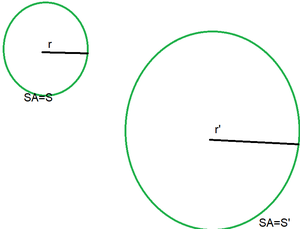### Question 10: A capsule of medicine is in the shape of a sphere of diameter 3.5mm. How much medicine (in mm3) is needed to fill this capsule? (Assume π = 22/7)

Solution:

Given: Diameter of capsule = 3.5 mm

Therefore, the radius of capsule, r = (3.5/2) mm = 1.75mm

By using the formula, volume of spherical capsule = 4/3 πr3

= (4/3)×(22/7)×(1.75)

= 22.458 mm3

Therefore, the volume of the spherical capsule is 22.46 mm3.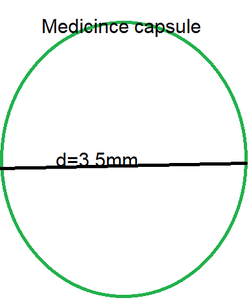My Personal Notes arrow_drop_up
Related Tutorials